# widebandTwoRayChannel

Wideband two-ray propagation channel

## Description

The widebandTwoRayChannel models a wideband two-ray propagation channel. A two-ray propagation channel is the simplest type of multipath channel. You can use a two-ray channel to simulate propagation of signals in a homogeneous, isotropic medium with a single reflecting boundary. This type of medium has two propagation paths: a line-of-sight (direct) propagation path from one point to another and a ray path reflected from the boundary.

You can use this System object™ for short-range radar and mobile communications applications where the signals propagate along straight paths and the earth is assumed to be flat. You can also use this object for sonar and microphone applications. For acoustic applications, you can choose nonpolarized fields and adjust the propagation speed to be the speed of sound in air or water. You can use widebandTwoRayChannel to model propagation from several points simultaneously.

Although the System object works for all frequencies, the attenuation models for atmospheric gases and rain are valid for electromagnetic signals in the frequency range 1–1000 GHz only. The attenuation model for fog and clouds is valid for 10–1000 GHz. Outside these frequency ranges, the System object uses the nearest valid value.

The widebandTwoRayChannel System object applies range-dependent time delays to the signals, as well as gains or losses, phase shifts, and boundary reflection loss. When either the source or destination is moving, the System object applies Doppler shifts to the signals.

Signals at the channel output can be kept separate or be combined. If you keep the signals separate, both signals arrive at the destination separately and are not combined. If you choose to combine the signals, the two signals from the source propagate separately but are coherently summed at the destination into a single quantity. Choose this option when the difference between the sensor or array gains in the directions of the two paths is insignificant.

In contrast to the phased.WidebandFreeSpace and phased.WidebandLOSChannel System objects, this System object does not support two-way propagation.

To compute the propagation delay for specified source and receiver points:

1. Define and set up your two-ray channel. See Creation.

2. Call the step method to compute the propagated signal using the properties of the widebandTwoRayChannel System object.

Note

Alternatively, instead of using the step method to perform the operation defined by the System object, you can call the object with arguments, as if it were a function. For example, y = step(obj,x) and y = obj(x) perform equivalent operations.

## Construction

channel = widebandTwoRayChannel creates a two-ray propagation channel System object, channel.

channel = widebandTwoRayChannel(Name,Value) creates a System object, channel, with each specified property Name set to the specified Value. You can specify additional name and value pair arguments in any order as (Name1,Value1,...,NameN,ValueN).

## Properties

expand all

Signal propagation speed, specified as a positive scalar. Units are in meters per second. The default propagation speed is the value returned by physconst('LightSpeed'). See physconst for more information.

Example: 3e8

Data Types: double

Operating frequency, specified as a positive scalar. Units are in Hz.

Example: 1e9

Data Types: double

Option to enable the atmospheric attenuation model, specified as a false or true. Set this property to true to add signal attenuation caused by atmospheric gases, rain, fog, or clouds. Set this property to false to ignore atmospheric effects in propagation.

Setting SpecifyAtmosphere to true, enables the Temperature, DryAirPressure, WaterVapourDensity, LiquidWaterDensity, and RainRate properties.

Data Types: logical

Ambient temperature, specified as a real-valued scalar. Units are in degrees Celsius.

Example: 20.0

#### Dependencies

To enable this property, set SpecifyAtmosphere to true.

Data Types: double

Atmospheric dry air pressure, specified as a positive real-valued scalar. Units are in pascals (Pa). The default value of this property corresponds to one standard atmosphere.

Example: 101.0e3

#### Dependencies

To enable this property, set SpecifyAtmosphere to true.

Data Types: double

Atmospheric water vapor density, specified as a positive real-valued scalar. Units are in g/m3.

Example: 7.4

#### Dependencies

To enable this property, set SpecifyAtmosphere to true.

Data Types: double

Liquid water density of fog or clouds, specified as a nonnegative real-valued scalar. Units are in g/m3. Typical values for liquid water density are 0.05 for medium fog and 0.5 for thick fog.

Example: 0.1

#### Dependencies

To enable this property, set SpecifyAtmosphere to true.

Data Types: double

Rainfall rate, specified as a nonnegative scalar. Units are in mm/hr.

Example: 10.0

#### Dependencies

To enable this property, set SpecifyAtmosphere to true.

Data Types: double

Sample rate of signal, specified as a positive scalar. Units are in Hz. The System object uses this quantity to calculate the propagation delay in units of samples.

Example: 1e6

Data Types: double

Number of processing subbands, specified as a positive integer.

Example: 128

Data Types: double

Option to enable polarized fields, specified as false or true. Set this property to true to enable polarization. Set this property to false to ignore polarization.

Data Types: logical

Ground reflection coefficient for the field at the reflection point, specified as a complex-valued scalar or a complex-valued 1-by-N row vector. Each coefficient has an absolute value less than or equal to one. The quantity N is the number of two-ray channels. Units are dimensionless. Use this property to model nonpolarized signals. To model polarized signals, use the GroundRelativePermittivity property.

Example: -0.5

#### Dependencies

To enable this property, set EnablePolarization to false.

Data Types: double
Complex Number Support: Yes

Relative permittivity of the ground at the reflection point, specified as a positive real-valued scalar or a 1-by-N real-valued row vector of positive values. The dimension N is the number of two-ray channels. Permittivity units are dimensionless. Relative permittivity is defined as the ratio of actual ground permittivity to the permittivity of free space. This property applies when you set the EnablePolarization property to true. Use this property to model polarized signals. To model nonpolarized signals, use the GroundReflectionCoefficient property.

Example: 5

#### Dependencies

To enable this property, set EnablePolarization to true.

Data Types: double

Option to combine the two rays at channel output, specified as true or false. When this property is true, the object coherently adds the line-of-sight propagated signal and the reflected path signal when forming the output signal. Use this mode when you do not need to include the directional gain of an antenna or array in your simulation.

Data Types: logical

Source of maximum one-way propagation distance, specified as 'Auto' or 'Property'. The maximum one-way propagation distance is used to allocate sufficient memory for signal delay computation. When you set this property to 'Auto', the System object automatically allocates memory. When you set this property to 'Property', you specify the maximum one-way propagation distance using the value of the MaximumDistance property.

Data Types: char

Maximum one-way propagation distance, specified as a positive real-valued scalar. Units are in meters. Any signal that propagates more than the maximum one-way distance is ignored. The maximum distance must be greater than or equal to the largest position-to-position distance.

Example: 5000

#### Dependencies

To enable this property, set the MaximumDistanceSource property to 'Property'.

Data Types: double

The source of the maximum number of samples of the input signal, specified as 'Auto' or 'Property'. When you set this property to 'Auto', the propagation model automatically allocates enough memory to buffer the input signal. When you set this property to 'Property', you specify the maximum number of samples in the input signal using the MaximumNumInputSamples property. Any input signal longer than that value is truncated.

To use this object with variable-size signals in a MATLAB® Function Block in Simulink®, set the MaximumNumInputSamplesSource property to 'Property' and set a value for the MaximumNumInputSamples property.

Example: 'Property'

#### Dependencies

To enable this property, set MaximumDistanceSource to 'Property'.

Data Types: char

Maximum number of input signal samples, specified as a positive integer. The size of the input signal is the number of rows in the input matrix. Any input signal longer than this number is truncated. To process signals completely, ensure that this property value is greater than any maximum input signal length.

The waveform-generating System objects determine the maximum signal size:

• For any waveform, if the waveform OutputFormat property is set to 'Samples', the maximum signal length is the value specified in the NumSamples property.

• For pulse waveforms, if the OutputFormat is set to 'Pulses', the signal length is the product of the smallest pulse repetition frequency, the number of pulses, and the sample rate.

• For continuous waveforms, if the OutputFormat is set to 'Sweeps', the signal length is the product of the sweep time, the number of sweeps, and the sample rate.

Example: 2048

#### Dependencies

To enable this property, set MaximumNumInputSamplesSource to 'Property'.

Data Types: double

## Methods

 reset Reset states of System object step Propagate wideband signal from point to point using two-ray channel model
Common to All System Objects
release

Allow System object property value changes

## Examples

collapse all

This example illustrates the two-ray propagation of a wideband signal, showing how the signals from the line-of-sight path and reflected path arrive at the receiver at different times.

Note: You can replace each call to the function with the equivalent step syntax. For example, replace myObject(x) with step(myObject,x).

Create and Plot Transmitted Waveform

Create a nonpolarized electromagnetic field consisting of two linear FM waveform pulses at a carrier frequency of 100 MHz. Assume the pulse width is 20 μs and the sampling rate is 10 MHz. The bandwidth of the pulse is 1 MHz. Assume a 50% duty cycle so that the pulse width is one-half the pulse repetition interval. Create a two-pulse wave train. Set the GroundReflectionCoefficient to –0.9 to model strong ground reflectivity. Propagate the field from a stationary source to a stationary receiver. The vertical separation of the source and receiver is approximately 10 km.

c = physconst('LightSpeed'); fs = 10e6; pw = 20e-6; pri = 2*pw; PRF = 1/pri; fc = 100e6; lambda = c/fc; bw = 1e6; waveform = phased.LinearFMWaveform('SampleRate',fs,'PulseWidth',pw,... 'PRF',PRF,'OutputFormat','Pulses','NumPulses',2,'SweepBandwidth',bw,... 'SweepDirection','Down','Envelope','Rectangular','SweepInterval',... 'Positive'); wav = waveform(); n = size(wav,1); plot([0:(n-1)]/fs*1e6,real(wav),'b') xlabel('Time (\mu s)') ylabel('Waveform Magnitude')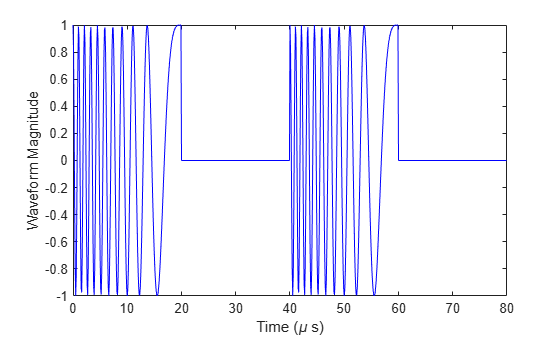Specify the Location of Source and Receiver

Place the source and receiver about 1 km apart horizontally and approximately 5 km apart vertically.

pos1 = [0;0;100]; pos2 = [1e3;0;5.0e3]; vel1 = [0;0;0]; vel2 = [0;0;0];

Create a Wideband Two-Ray Channel System Object

Create a two-ray propagation channel System object™ and propagate the signal along both the line-of-sight and reflected ray paths. The same signal is propagated along both paths.

channel = widebandTwoRayChannel('SampleRate',fs,... 'GroundReflectionCoefficient',-0.9,'OperatingFrequency',fc,... 'CombinedRaysOutput',false); prop_signal = channel([wav,wav],pos1,pos2,vel1,vel2); [rng2,angs] = rangeangle(pos2,pos1,'two-ray');

Calculate time delays in μs.

tm = rng2/c*1e6; disp(tm)
 16.6815 17.3357 

Display the calculated propagation paths azimuth and elevation angles in degrees.

disp(angs)
 0 0 78.4654 -78.9063 

Plot the Propagated Signals

1. Plot the real part of the signal propagated along the line-of-sight path.

2. Plot the real part of the signal propagated along the reflected path.

3. Plot the real part of the coherent sum of the two signals.

n = size(prop_signal,1); delay = [0:(n-1)]/fs*1e6; subplot(3,1,1) plot(delay,real([prop_signal(:,1)]),'b') grid xlabel('Time (\mu sec)') ylabel('Real Part') title('Direct Path') subplot(3,1,2) plot(delay,real([prop_signal(:,2)]),'b') grid xlabel('Time (\mu sec)') ylabel('Real Part') title('Reflected Path') subplot(3,1,3) plot(delay,real([prop_signal(:,1) + prop_signal(:,2)]),'b') grid xlabel('Time (\mu sec)') ylabel('Real Part') title('Combined Paths')The delay of the reflected path signal agrees with the predicted delay. The magnitude of the coherently combined signal is less than either of the propagated signals. This result indicates that the two signals contain some interference.

Compute the result of propagating a wideband LFM signal in a two-ray environment from a radar 10 meters above the origin (0,0,10) to a target at (3000,2000,2000) meters. Assume that the radar and target are stationary and that the transmitting antenna is isotropic. Combine the signal from the two paths and compare the signal to a signal propagating in free space. The system operates at 300 MHz. Set the CombinedRaysOutput property to true to combine the direct path and reflected path signals when forming the output signal.

Note: This example runs only in R2016b or later. If you are using an earlier release, replace each call to the function with the equivalent step syntax. For example, replace myObject(x) with step(myObject,x).

Create a linear FM waveform.

fop = 300.0e6; fs = 1.0e6; waveform = phased.LinearFMWaveform(); x = waveform();

Specify the target position and velocity.

posTx = [0; 0; 10]; posTgt = [3000; 2000; 2000]; velTx = [0;0;0]; velTgt = [0;0;0];

Model the free space propagation.

fschannel = phased.WidebandFreeSpace('SampleRate',waveform.SampleRate); y_fs = fschannel(x,posTx,posTgt,velTx,velTgt);

Model two-ray propagation from the position of the radar to the target.

tworaychannel = widebandTwoRayChannel('SampleRate',waveform.SampleRate,... 'CombinedRaysOutput',true); y_tworay = tworaychannel(x,posTx,posTgt,velTx,velTgt); plot(abs([y_tworay y_fs])) legend('Wideband two-ray (Position 1)','Wideband free space (Position 1)',... 'Location','best') xlabel('Samples') ylabel('Signal Magnitude') hold on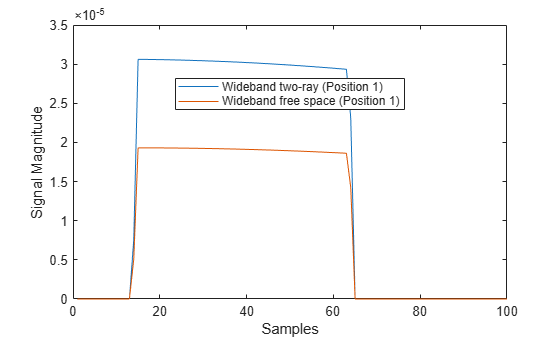Move the radar by 10 meters horizontally to a second position.

posTx = posTx + [10;0;0]; y_fs = fschannel(x,posTx,posTgt,velTx,velTgt); y_tworay = tworaychannel(x,posTx,posTgt,velTx,velTgt); plot(abs([y_tworay y_fs])) legend('Wideband two-ray (Position 1)','Wideband free space (Position 1)',... 'Wideband two-ray (Position 2)','Wideband free space (Position 2)',... 'Location','best') hold off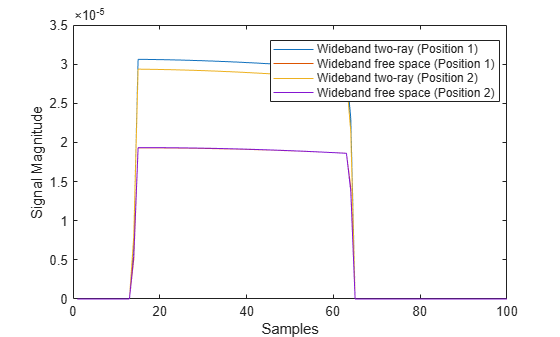The free-space propagation losses are the same for both the first and second positions of the radar. The two-ray losses are different due to the interference effect of the two-ray paths.

Create a polarized electromagnetic field consisting of linear FM waveform pulses. Propagate the field from a stationary source with a crossed-dipole antenna element to a stationary receiver approximately 10 km away. The transmitting antenna is 100 m above the ground. The receiving antenna is 150 m above the ground. The receiving antenna is also a crossed-dipole. Plot the received signal.

Note: You can replace each call to the function with the equivalent step syntax. For example, replace myObject(x) with step(myObject,x).

Assume the pulse width is$10\mu s$ and the sampling rate is 10 MHz. The bandwidth of the pulse is 1 MHz. Assume a 50% duty cycle in which the pulse width is one-half the pulse repetition interval. Create a two-pulse wave train. Assume a carrier frequency of 100 MHz.

c = physconst('LightSpeed'); fs = 20e6; pw = 10e-6; pri = 2*pw; PRF = 1/pri; fc = 100e6; bw = 1e6; lambda = c/fc;

Set Up Required System Objects

Use a GroundRelativePermittivity of 10.

waveform = phased.LinearFMWaveform('SampleRate',fs,'PulseWidth',pw,... 'PRF',PRF,'OutputFormat','Pulses','NumPulses',2,'SweepBandwidth',bw,... 'SweepDirection','Down','Envelope','Rectangular','SweepInterval',... 'Positive'); antenna = phased.CrossedDipoleAntennaElement(... 'FrequencyRange',[50,200]*1e6); radiator = phased.Radiator('Sensor',antenna,'OperatingFrequency',fc,... 'Polarization','Combined'); channel = phased.WidebandTwoRayChannel('SampleRate',fs,... 'OperatingFrequency',fc,'CombinedRaysOutput',false,... 'EnablePolarization',true,'GroundRelativePermittivity',10); collector = phased.Collector('Sensor',antenna,'OperatingFrequency',fc,... 'Polarization','Combined');

Set Up Scene Geometry

Specify transmitter and receiver positions, velocities, and orientations. Place the source and receiver approximately 1000 m apart horizontally and approximately 50 m apart vertically.

posTx = [0;100;100]; posRx = [1000;0;150]; velTx = [0;0;0]; velRx = [0;0;0]; laxRx = rotz(180); laxTx = rotx(1)*eye(3);

Create and Radiate Signals from Transmitter

Compute the transmission angles for the two rays traveling toward the receiver. These angles are defined with respect to the transmitter local coordinate system. The phased.Radiator System object(TM) uses these angles to apply separate antenna gains to the two signals.

[rng,angsTx] = rangeangle(posRx,posTx,laxTx,'two-ray'); wav = waveform();

Plot the transmitted waveform.

n = size(wav,1); plot([0:(n-1)]/fs*1000000,real(wav)) xlabel('Time ({\mu}sec)') ylabel('Waveform')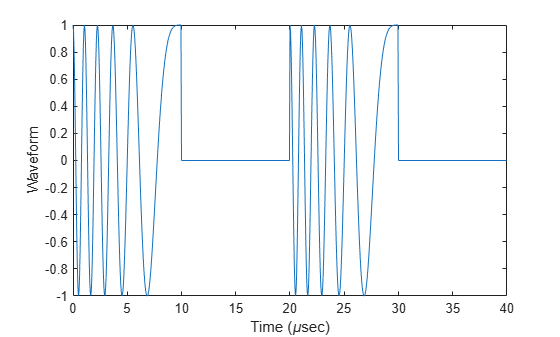sig = radiator(wav,angsTx,laxTx);

Propagate the signals to the receiver via a two-ray channel.

prop_sig = channel(sig,posTx,posRx,velTx,velRx);

Compute the reception angles for the two rays arriving at the receiver. These angles are defined with respect to the receiver local coordinate system. The phased.Collector System object(TM) uses these angles to apply separate antenna gains to the two signals.

[rng1,angsRx] = rangeangle(posTx,posRx,laxRx,'two-ray'); delays = rng1/c*1e6
delays = 1×2 3.3564 3.4544 

Collect and combine the received rays.

y = collector(prop_sig,angsRx,laxRx);

plot([0:(n-1)]/fs*1000000,real(y)) xlabel('Time ({\mu}sec)') ylabel('Received Waveform')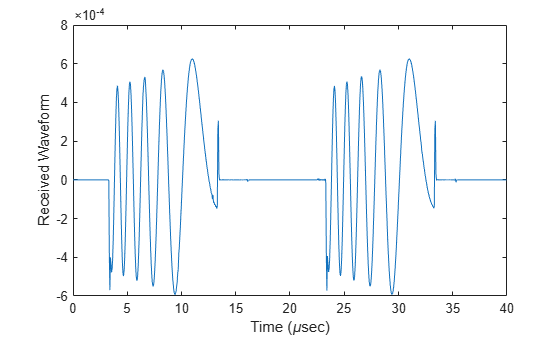Propagate a wideband linear FM signal in a two-ray channel. The signal bandwidth is 15% of the carrier frequency. Assume there is signal loss caused by atmospheric gases and rain. The signal propagates from a transmitter located at (0,0,0) meters in the global coordinate system to a receiver at (10000,200,30) meters. Assume that the transmitter and the receiver are stationary and that they both have cosine antenna patterns. Plot the received signal. Set the dry air pressure to 102.0 Pa and the rain rate to 5 mm/hr.

c = physconst('LightSpeed'); fs = 40e6; pw = 10e-6; pri = 2.5*pw; PRF = 1/pri; fc = 100e6; bw = 15e6; lambda = c/fc;

Create the required System objects.

waveform = phased.LinearFMWaveform('SampleRate',fs,'PulseWidth',pw,... 'PRF',PRF,'OutputFormat','Pulses','NumPulses',2,'SweepBandwidth',bw,... 'SweepDirection','Down','Envelope','Rectangular','SweepInterval',... 'Positive'); antenna = phased.CosineAntennaElement; radiator = phased.Radiator('Sensor',antenna); collector = phased.Collector('Sensor',antenna); channel = widebandTwoRayChannel('SampleRate',waveform.SampleRate,... 'CombinedRaysOutput',false,'GroundReflectionCoefficient',0.95,... 'SpecifyAtmosphere',true,'Temperature',20,... 'DryAirPressure',102.5,'RainRate',5.0);

Set up the scene geometry. Specify transmitter and receiver positions and velocities. The transmitter and receiver are stationary.

posTx = [0;0;0]; posRx = [10000;200;30]; velTx = [0;0;0]; velRx = [0;0;0];

Specify the transmitting and receiving radar antenna orientations with respect to the global coordinates. The transmitting antenna points along the positive x-direction and the receiving antenna points close to the negative x-direction.

laxTx = eye(3); laxRx = rotx(5)*rotz(170);

Compute the transmission angles which are the angles at which the two rays traveling toward the receiver leave the transmitter. The phased.Radiator System object™ uses these angles to apply separate antenna gains to the two signals. Because the antenna gains depend on path direction, you must transmit and receive the two rays separately.

[~,angTx] = rangeangle(posRx,posTx,laxTx,'two-ray');

Create and Radiate Signals from Transmitter

Radiate the signals along the transmission directions.

wavfrm = waveform(); wavtrans = radiator(wavfrm,angTx);

Propagate the signals to the receiver via a two-ray channel.

wavrcv = channel(wavtrans,posTx,posRx,velTx,velRx);

Compute the angle at which the two rays traveling from the transmitter arrive at the receiver. The phased.Collector System object™ uses these angles to apply separate antenna gains to the two signals.

[~,angRcv] = rangeangle(posTx,posRx,laxRx,'two-ray');

Collect and combine the two received rays.

yR = collector(wavrcv,angRcv);

dt = 1/waveform.SampleRate; n = size(yR,1); plot([0:(n-1)]*dt*1e6,real(yR)) xlabel('Time ({\mu}sec)') ylabel('Signal Magnitude')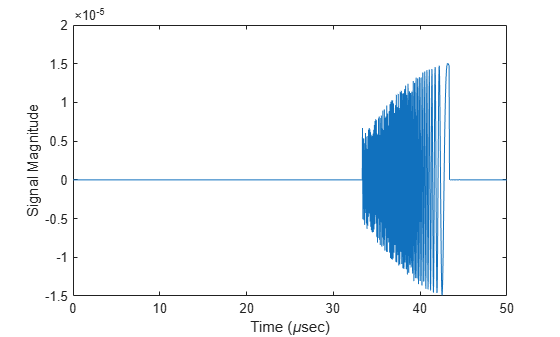expand all

## References

 Proakis, J. Digital Communications. New York: McGraw-Hill, 2001.

 Skolnik, M. Introduction to Radar Systems, 3rd Ed. New York: McGraw-Hill.

 Saakian, A. Radio Wave Propagation Fundamentals. Norwood, MA: Artech House, 2011.

 Balanis, C. Advanced Engineering Electromagnetics. New York: Wiley & Sons, 1989.

 Rappaport, T. Wireless Communications: Principles and Practice, 2nd Ed New York: Prentice Hall, 2002.

 Radiocommunication Sector of the International Telecommunication Union. Recommendation ITU-R P.676-10: Attenuation by atmospheric gases. 2013.

 Radiocommunication Sector of the International Telecommunication Union. Recommendation ITU-R P.840-6: Attenuation due to clouds and fog. 2013.

 Radiocommunication Sector of the International Telecommunication Union. Recommendation ITU-R P.838-3: Specific attenuation model for rain for use in prediction methods. 2005.

## Version History

Introduced in R2021a